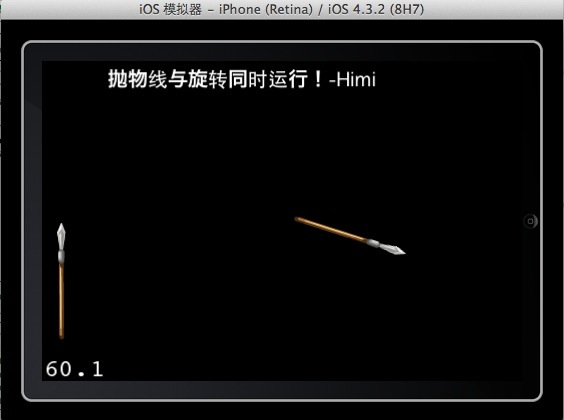【iOS-Cocos2d游戏开发之十三】CCSprite利用Bezier（贝塞尔）做抛物线动作并让CCSprite同时播放两个Action动作！

年薪达不到25W大数据工程师、拿不到Offer全额退款->>>//  抛物线    -Himi
//mSprite：需要做抛物线的精灵
//startPoint:起始位置
//endPoint:中止位置
//dirTime:起始位置到中止位置的所需时间
- (void) moveWithParabola:(CCSprite*)mSprite startP:(CGPoint)startPoint endP:(CGPoint)endPoint dirTime:(float)time{
float sx = startPoint.x;
float sy = startPoint.y;
float ex =endPoint.x+50;
float ey =endPoint.y+150;
int h = [mSprite contentSize].height*0.5;
ccBezierConfig bezier; // 创建贝塞尔曲线
bezier.controlPoint_1 = ccp(sx, sy); // 起始点
bezier.controlPoint_2 = ccp(sx+(ex-sx)*0.5, sy+(ey-sy)*0.5+200); //控制点
bezier.endPosition = ccp(endPoint.x-30, endPoint.y+h); // 结束位置
CCBezierTo *actionMove = [CCBezierTo actionWithDuration:time bezier:bezier];
[mSprite runAction:actionMove];
}

//----  OK，下面来介绍第二个知识点：

这里为了讲解方便，我在刚才封装的贝塞尔曲线上的方法上进行修改，添加一个旋转的动作，让贝塞尔曲线运动与旋转动作同时播放，这里Himi使用一根“矛”的图片作用示意图来说明，因为矛与箭的运动方式最常用的动作肯定是一边旋转一边抛物线位移，这样才更加真实；

OK，看下封装角度后的抛物线并同时旋转方法如下:

//  抛物线运动并同时旋转    -Himi
//mSprite：需要做抛物线的精灵
//startPoint:起始位置
//endPoint:中止位置
//startA:起始角度
//endA:中止角度
//dirTime:起始位置到中止位置的所需时间
- (void) moveWithParabola:(CCSprite*)mSprite startP:(CGPoint)startPoint endP:(CGPoint)endPoint startA:(float)startAngle endA:(float)endAngle dirTime:(float)time{
float sx = startPoint.x;
float sy = startPoint.y;
float ex =endPoint.x+50;
float ey =endPoint.y+150;
int h = [mSprite contentSize].height*0.5;
//设置精灵的起始角度
sprite.rotation=startAngle;
ccBezierConfig bezier; // 创建贝塞尔曲线
bezier.controlPoint_1 = ccp(sx, sy); // 起始点
bezier.controlPoint_2 = ccp(sx+(ex-sx)*0.5, sy+(ey-sy)*0.5+200); //控制点
bezier.endPosition = ccp(endPoint.x-30, endPoint.y+h); // 结束位置
CCBezierTo *actionMove = [CCBezierTo actionWithDuration:time bezier:bezier];
//创建精灵旋转的动作
CCRotateTo *actionRotate =[CCRotateTo actionWithDuration:time angle:endAngle];
//将两个动作封装成一个同时播放进行的动作
CCAction * action = [CCSpawn actions:actionMove, actionRotate, nil];
[mSprite runAction:action];
}